Revolution in a clock

Chapter 5 Class 6 Understanding Elementary Shapes
Concept wise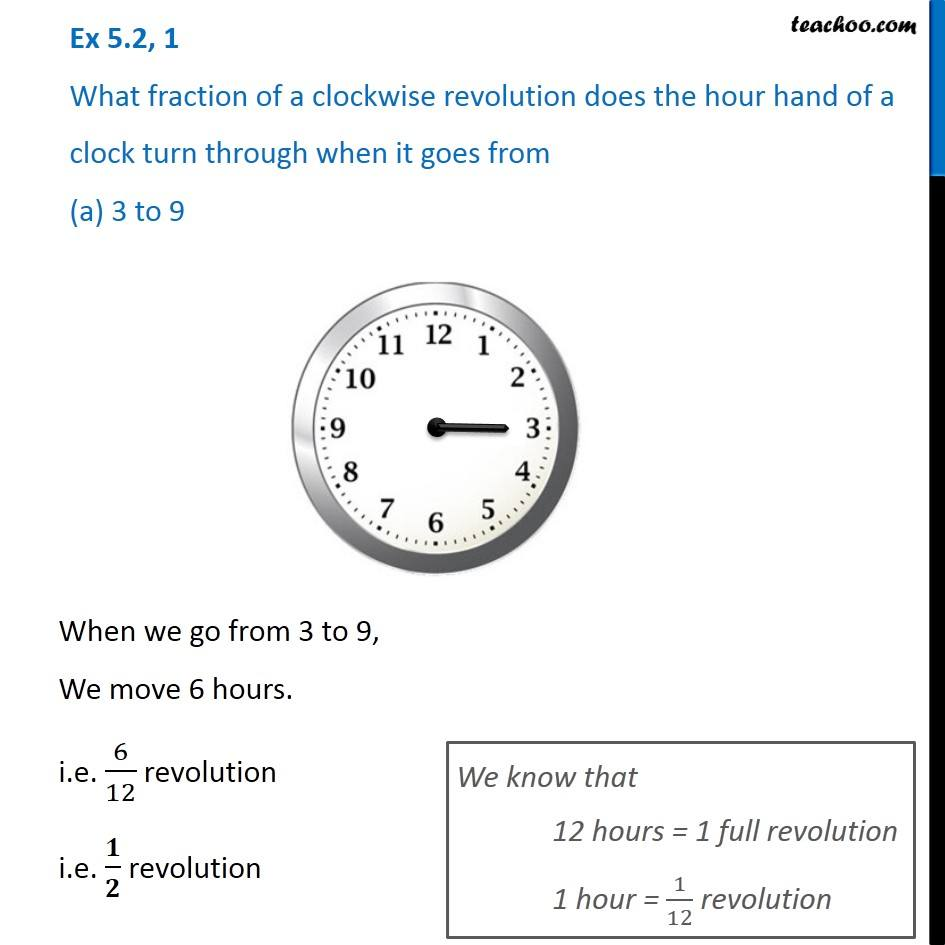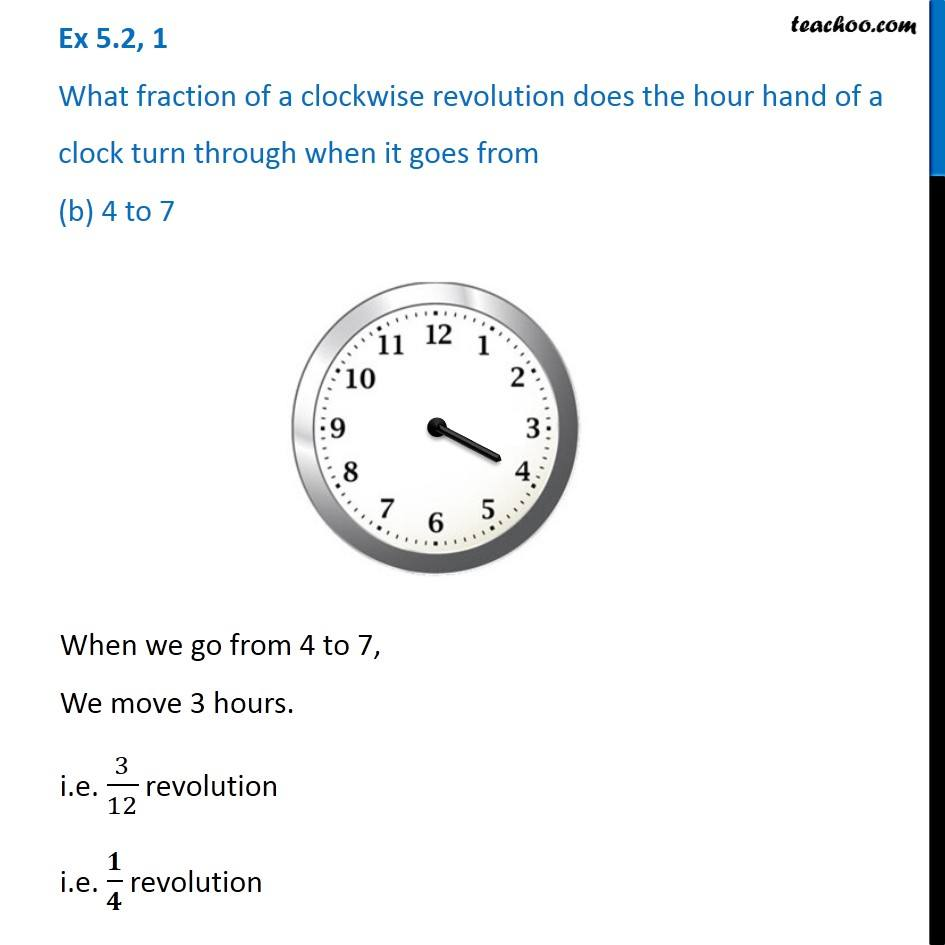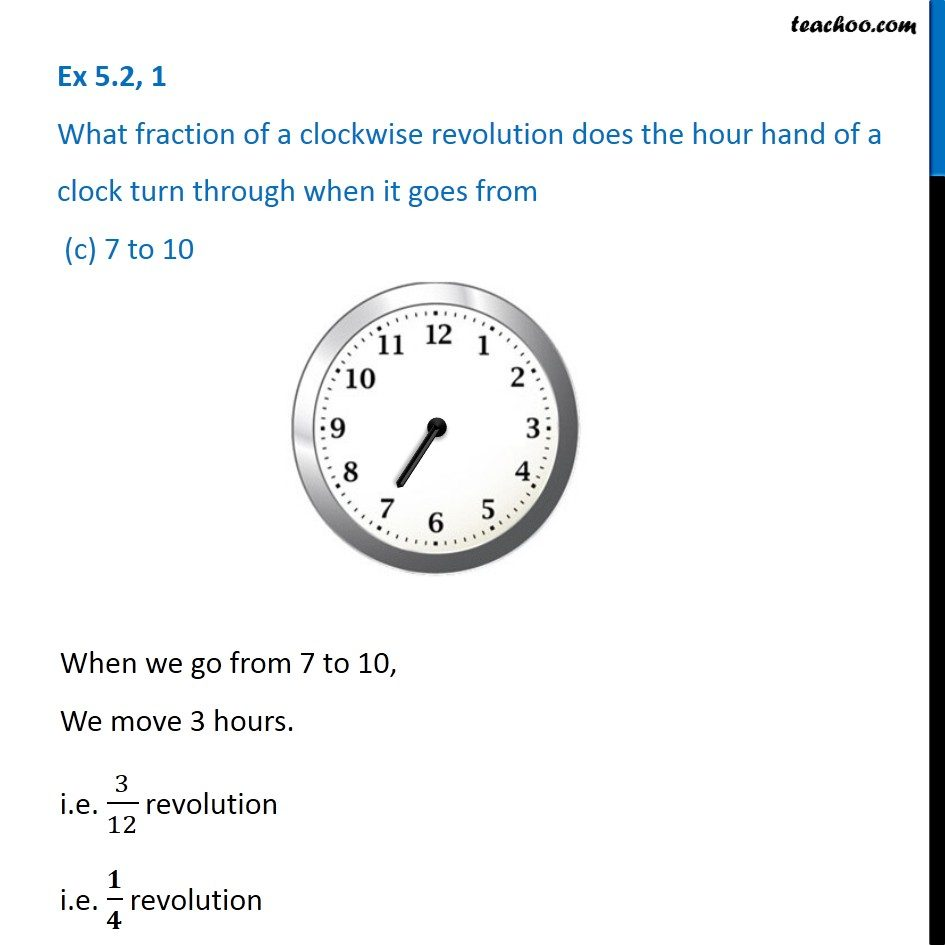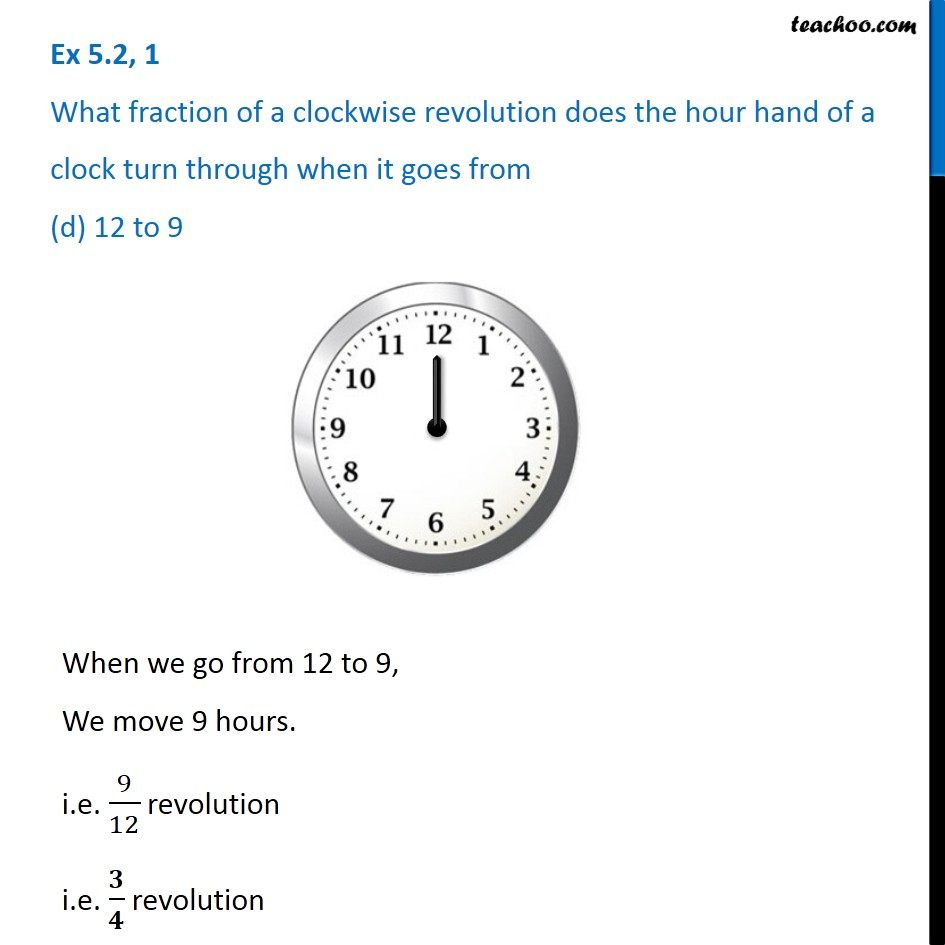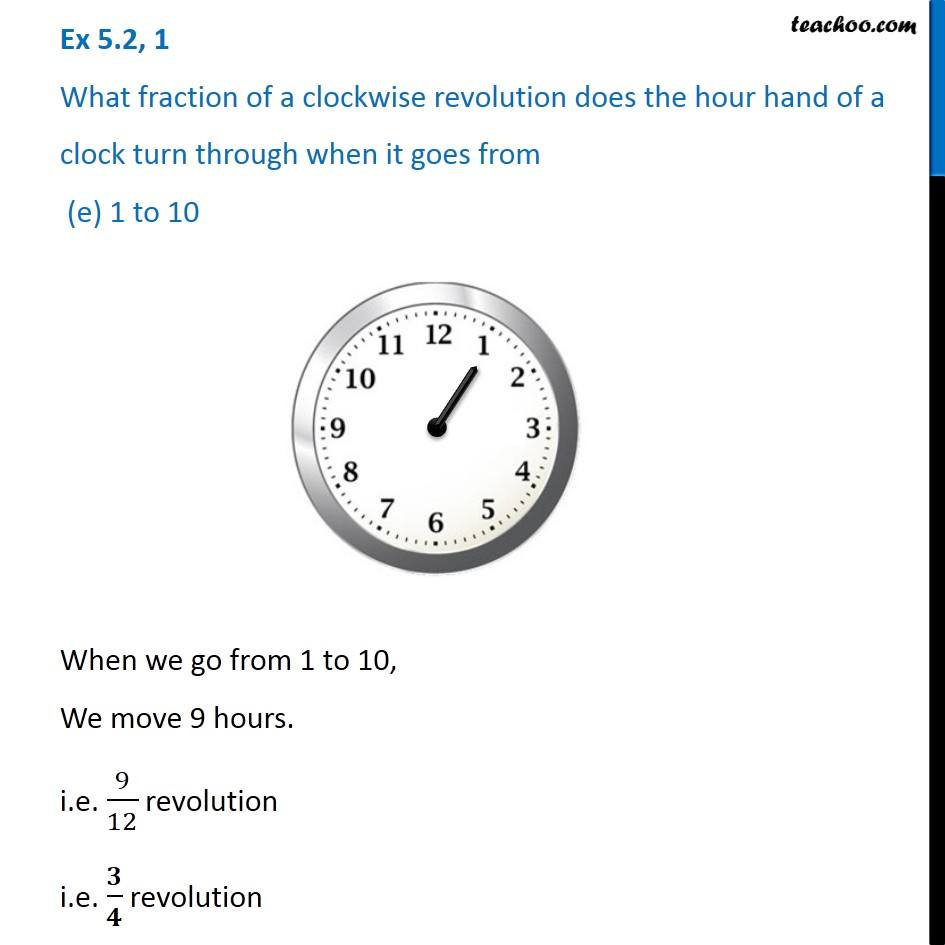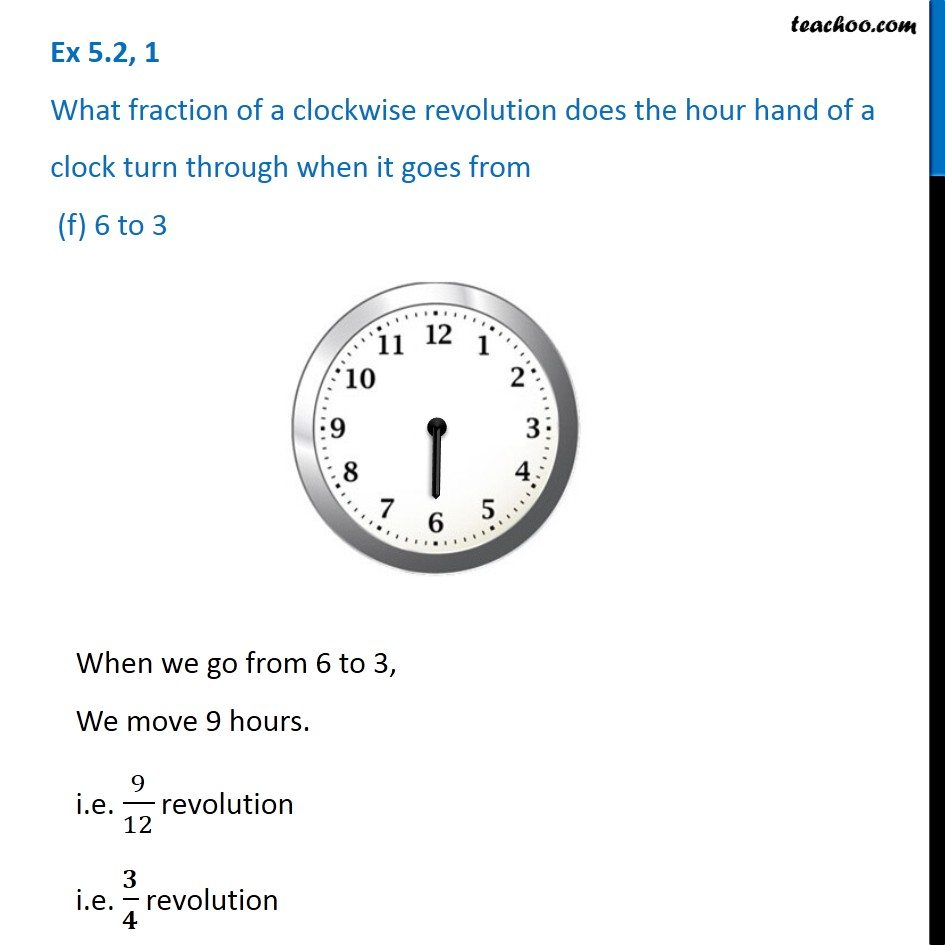Learn in your speed, with individual attention - Teachoo Maths 1-on-1 Class

### Transcript

Ex 5.2, 1 What fraction of a clockwise revolution does the hour hand of a clock turn through when it goes from (a) 3 to 9 When we go from 3 to 9, We move 6 hours. i.e. 6/12 revolution i.e. 𝟏/𝟐 revolution We know that 12 hours = 1 full revolution 1 hour = 1/12 revolution Ex 5.2, 1 What fraction of a clockwise revolution does the hour hand of a clock turn through when it goes from (b) 4 to 7 When we go from 4 to 7, We move 3 hours. i.e. 3/12 revolution i.e. 𝟏/𝟒 revolution Ex 5.2, 1 What fraction of a clockwise revolution does the hour hand of a clock turn through when it goes from (c) 7 to 10 When we go from 7 to 10, We move 3 hours. i.e. 3/12 revolution i.e. 𝟏/𝟒 revolution Ex 5.2, 1 What fraction of a clockwise revolution does the hour hand of a clock turn through when it goes from (d) 12 to 9 When we go from 12 to 9, We move 9 hours. i.e. 9/12 revolution i.e. 𝟑/𝟒 revolution Ex 5.2, 1 What fraction of a clockwise revolution does the hour hand of a clock turn through when it goes from (e) 1 to 10 When we go from 1 to 10, We move 9 hours. i.e. 9/12 revolution i.e. 𝟑/𝟒 revolution Ex 5.2, 1 What fraction of a clockwise revolution does the hour hand of a clock turn through when it goes from (f) 6 to 3 When we go from 6 to 3, We move 9 hours. i.e. 9/12 revolution i.e. 𝟑/𝟒 revolution